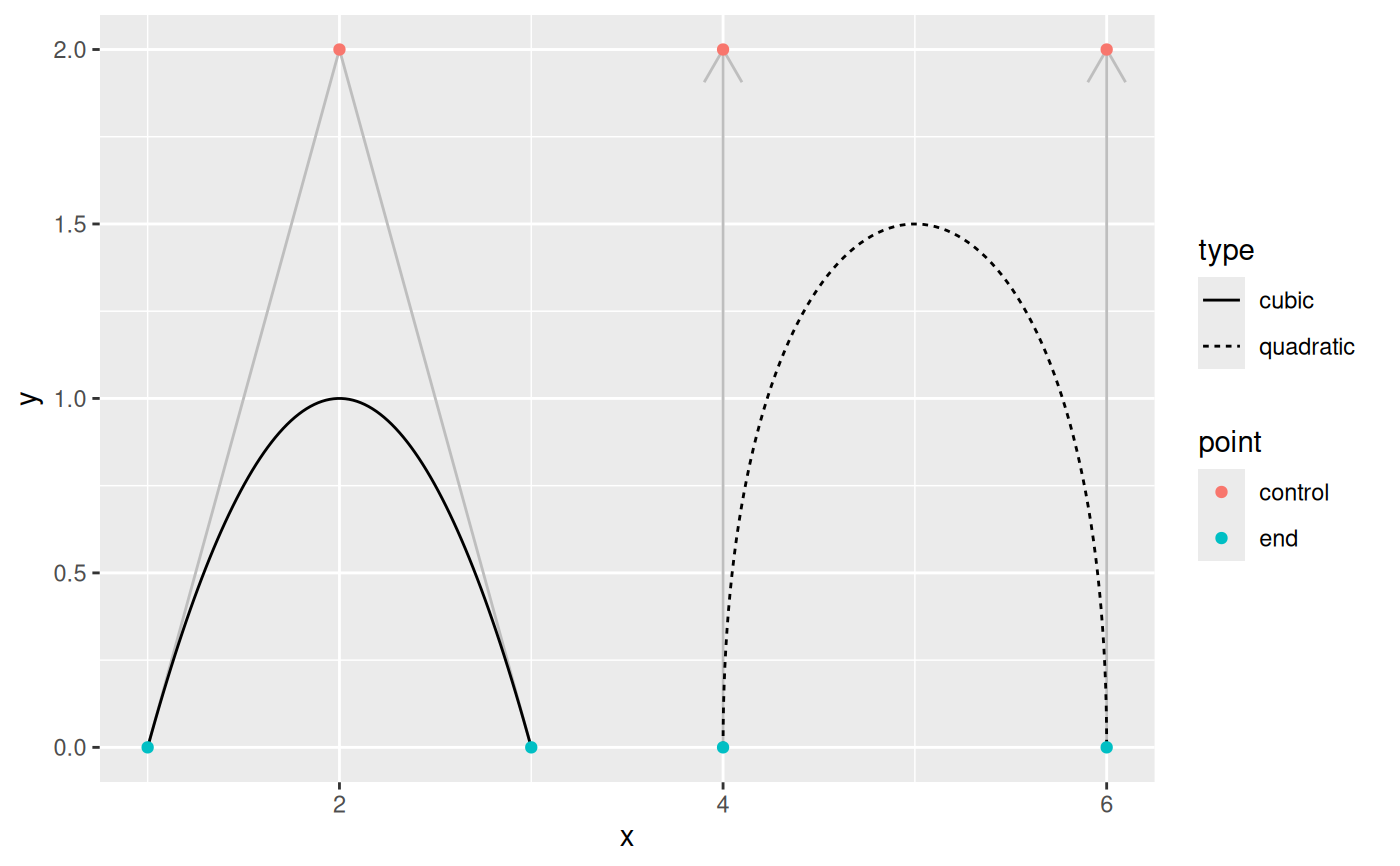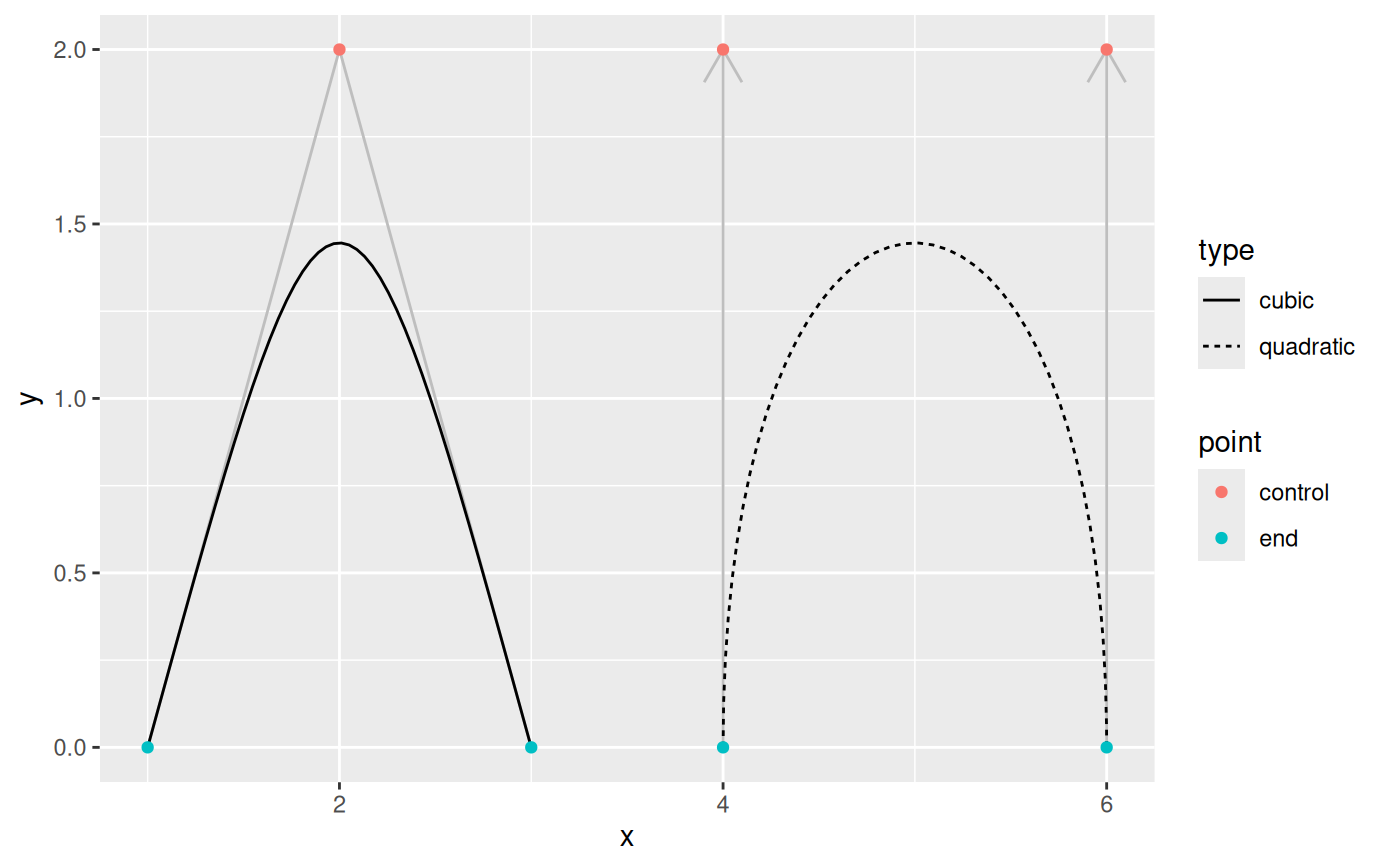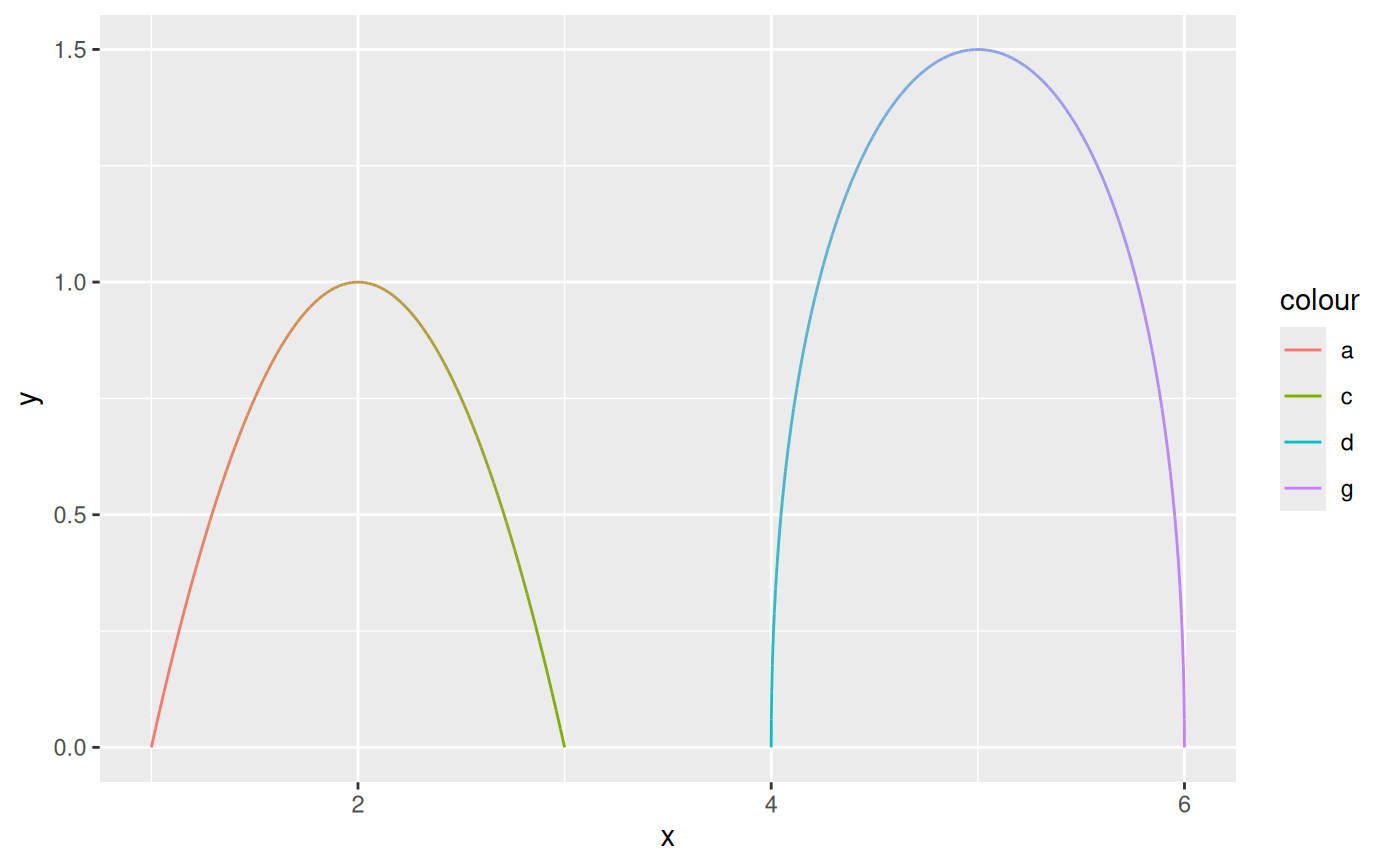This set of geoms makes it possible to connect points creating either quadratic or cubic beziers. bezier and bezier2 both work by calculating points along the bezier and connecting these to draw the curve. bezier0 directly draws the bezier using bezierGrob. In line with the geom_link() and geom_link2() differences geom_bezier creates the points, assign an index to each interpolated point and repeat the aesthetics for the start point, while geom_bezier2 interpolates the aesthetics between the start and end points.

stat_bezier(
mapping = NULL,
data = NULL,
geom = "path",
position = "identity",
na.rm = FALSE,
show.legend = NA,
n = 100,
inherit.aes = TRUE,
...
)

geom_bezier(
mapping = NULL,
data = NULL,
stat = "bezier",
position = "identity",
arrow = NULL,
lineend = "butt",
na.rm = FALSE,
show.legend = NA,
inherit.aes = TRUE,
n = 100,
...
)

stat_bezier2(
mapping = NULL,
data = NULL,
geom = "path_interpolate",
position = "identity",
na.rm = FALSE,
show.legend = NA,
n = 100,
inherit.aes = TRUE,
...
)

geom_bezier2(
mapping = NULL,
data = NULL,
stat = "bezier2",
position = "identity",
arrow = NULL,
lineend = "butt",
na.rm = FALSE,
show.legend = NA,
inherit.aes = TRUE,
n = 100,
...
)

stat_bezier0(
mapping = NULL,
data = NULL,
geom = "bezier0",
position = "identity",
na.rm = FALSE,
show.legend = NA,
inherit.aes = TRUE,
...
)

geom_bezier0(
mapping = NULL,
data = NULL,
stat = "bezier0",
position = "identity",
arrow = NULL,
lineend = "butt",
na.rm = FALSE,
show.legend = NA,
inherit.aes = TRUE,
...
)

## Arguments

mapping

Set of aesthetic mappings created by aes(). If specified and inherit.aes = TRUE (the default), it is combined with the default mapping at the top level of the plot. You must supply mapping if there is no plot mapping.

data

The data to be displayed in this layer. There are three options:

If NULL, the default, the data is inherited from the plot data as specified in the call to ggplot().

A data.frame, or other object, will override the plot data. All objects will be fortified to produce a data frame. See fortify() for which variables will be created.

A function will be called with a single argument, the plot data. The return value must be a data.frame, and will be used as the layer data. A function can be created from a formula (e.g. ~ head(.x, 10)).

geom

The geometric object to use to display the data, either as a ggproto Geom subclass or as a string naming the geom stripped of the geom_ prefix (e.g. "point" rather than "geom_point")

position

Position adjustment, either as a string naming the adjustment (e.g. "jitter" to use position_jitter), or the result of a call to a position adjustment function. Use the latter if you need to change the settings of the adjustment.

na.rm

If FALSE, the default, missing values are removed with a warning. If TRUE, missing values are silently removed.

show.legend

logical. Should this layer be included in the legends? NA, the default, includes if any aesthetics are mapped. FALSE never includes, and TRUE always includes. It can also be a named logical vector to finely select the aesthetics to display.

n

The number of points to create for each segment

inherit.aes

If FALSE, overrides the default aesthetics, rather than combining with them. This is most useful for helper functions that define both data and aesthetics and shouldn't inherit behaviour from the default plot specification, e.g. borders().

...

Other arguments passed on to layer(). These are often aesthetics, used to set an aesthetic to a fixed value, like colour = "red" or size = 3. They may also be parameters to the paired geom/stat.

stat

The statistical transformation to use on the data for this layer, either as a ggproto Geom subclass or as a string naming the stat stripped of the stat_ prefix (e.g. "count" rather than "stat_count")

arrow

Arrow specification, as created by grid::arrow().

lineend

Line end style (round, butt, square).

## Details

Input data is understood as a sequence of data points the first being the start point, then followed by one or two control points and then the end point. More than 4 and less than 3 points per group will throw an error. grid::bezierGrob() only takes cubic beziers so if three points are supplied the middle one as duplicated. This, along with the fact that grid::bezierGrob() estimates the curve using an x-spline means that the curves produced by geom_bezier and geom_bezier2 deviates from those produced by geom_bezier0. If you want true bezier paths use geom_bezier or geom_bezier2.

## Aesthetics

geom_bezier, geom_bezier2 and geom_bezier0 understand the following aesthetics (required aesthetics are in bold):

• x

• y

• color

• linewidth

• linetype

• alpha

• lineend

## Computed variables

x, y

The interpolated point coordinates

index

The progression along the interpolation mapped between 0 and 1

## Examples

beziers <- data.frame(
x = c(1, 2, 3, 4, 4, 6, 6),
y = c(0, 2, 0, 0, 2, 2, 0),
type = rep(c('cubic', 'quadratic'), c(3, 4)),
point = c('end', 'control', 'end', 'end', 'control', 'control', 'end'),
colour = letters[1:7]
)
help_lines <- data.frame(
x = c(1, 3, 4, 6),
xend = c(2, 2, 4, 6),
y = 0,
yend = 2
)

# See how control points affect the bezier
ggplot() +
geom_segment(aes(x = x, xend = xend, y = y, yend = yend),
data = help_lines,
arrow = arrow(length = unit(c(0, 0, 0.5, 0.5), 'cm')),
colour = 'grey') +
geom_bezier(aes(x = x, y = y, group = type, linetype = type),
data = beziers) +
geom_point(aes(x = x, y = y, colour = point),
data = beziers)# geom_bezier0 is less exact
ggplot() +
geom_segment(aes(x = x, xend = xend, y = y, yend = yend),
data = help_lines,
arrow = arrow(length = unit(c(0, 0, 0.5, 0.5), 'cm')),
colour = 'grey') +
geom_bezier0(aes(x = x, y = y, group = type, linetype = type),
data = beziers) +
geom_point(aes(x = x, y = y, colour = point),
data = beziers)# Use geom_bezier2 to interpolate between endpoint aesthetics
ggplot(beziers) +
geom_bezier2(aes(x = x, y = y, group = type, colour = colour))